# Weights

Marry and John together weighing 49 kg. Their weights are in ratio 1:6. Determine their weights.

Correct result:

m =  7 kg
j =  42 kg

#### Solution:

m+j = 49
6m=1j

m+j = 49
6•m=1•j

j+m = 49
j-6m = 0

j = 42
m = 7

Our linear equations calculator calculates it.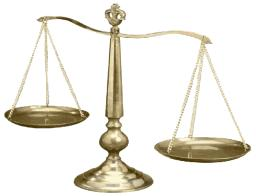We would be pleased if you find an error in the word problem, spelling mistakes, or inaccuracies and send it to us. Thank you!Tips to related online calculators
Do you have a system of equations and looking for calculator system of linear equations?

## Next similar math problems:

• HomeworkMother and Kate together weigh 24.5 kg more than the father. Father weighs 7.6 kg more than mother. Father weight 44.8 kg more than Kate. Determine weights of father mother and Kate.
• Bags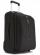The first bag has a weight of 18 kg, the second bag is a one-third lighter than the first and the third bag has three times less the weight of all three bags together. Determine their weights.
• Tea blendTea blends are maked from two kinds of tea. In standard tea mixture are two teas in the ratio 1:3 and 40 g costs 42 CZK. In the premium tea mixture are weighing two teas in the ratio 1:1 and 50 grams costs 60 CZK. How much cost 10 grams of more expensive
• Shoes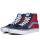Peter decided to measure shoes size of his three friends. Measured accurately and wrote as follows: Venda and Jenda together 52 cm Jenda and Zdenda together 58 cm Zdenda and Venda together 54 cm a) How long measures Vendy, Jenda and Zdenda shoes together?
• Purchase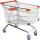Mother bought 5 boxes of milk and 7 kg of potatoes and paid a total CZK 147. Aunt bought 7 boxes of milk and 3 kg of potatoes and paid 131 CZK. What is the price of one carton of milk and 1 kg of potatoes? How CZK together would have saved if bought at th
• MeatHalf a kilogram of pork and three-quarters of a kilogram of beef cost total 5.1 USD. One quarter of a kilogram of beef and 1 kilogram of pork cost 4.9 USD. Determine the price of 1 kg of each type of meat.
• Chickens and rabbitsIn the yard were chickens and rabbits. Together they had 27 heads and 86 legs. How many chickens and how many rabbits were in the yard?
• ExcavationMr. Billy calculated that excavation for a water connection dig for 12 days. His friend would take 10 days. Billy worked 3 days alone. Then his friend came to help and started on the other end. On what day since the beginning of excavation they met?
• Trousers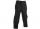Jarek bought new trousers, but the trousers were too long. Their length was in the ratio 5: 8 to Jarek height. Mother his trousers cut by 4 cm, thus the original ratio decreased by 4%. Determine Jarek's high.
• Children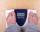Margaret and Zdena weighs the same and Petra 3 kg more. Together weigh 156 kg. How much does they weigh?
• Seamstress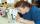Seamstress bought two kinds of textile together 25 meters. One at 50 SKK and the second 70 SKK per meter. How many meter bought from this two textiles when paid totally 1540 SKK?
• Revenue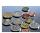Mr. Robert and Mr. Norbert get together € 1400. The revenue split in the ratio of 4:3, depending upon each work. How many got each of them?
• Three friends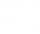Peter is eight times older than Rado, Rado is 3 times younger than Joseph. Together they have 120 years. Determine how many are each of them.
• EurosPeter, Jane and Thomas have together € 550. Tomas has 20 euros more than Jane, Peter € 150 less than Thomas. Determine how much has each of them.
• Motion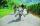Cyclist started at 9:00 from point S to point T. After 10 minutes, followed him at the same speed the second cyclist. Walker, which went from T to S, started at 9:35. After 71 minutes he met the first cyclist and after next 8 minutes the second cyclist. D
• Reservoir + waterReservoir completely filled with water weighs 12 kg. After pouring off three quarters of the amount of water weights 3 kg. Calculate the weight and volume of the reservoir.
• Coins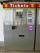In ticket maschine were together one hundred coins. They were only 20 and 50 cent coins. The sum total was 29 euros and 60 cents. How many were in ticket maschine coins and which type?